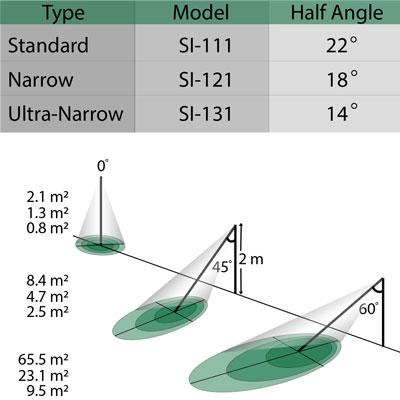# IRR Calculators### Calculate Field of View

The FOV determines the size of the target area viewed by the infrared radiometer. This is influenced by the height and angle at which the infrared radiometer sensor is mounted. Mounting at an angle can be useful; however, at certain angles the sky or distant objects will enter the field of view. For more detailed information concerning this subject, visit our post about Field of View. To use the calculator, simply enter your IRR viewing height (H), viewing angle (θ, relative to nadir view = 0°), and chose a sensor, then click calculate.

IRR viewing angle, θ (deg.):

IRR viewing height, H (m):

Sensor Selection:
SI-111SI-121SI-131SI-1H1

## Results

Error
Sensor:
IRR viewing angle, θ (deg.):
IRR viewing height, H (m):
Field of View, FOV (m²):

### Calculate Canopy Fraction

Fraction of Canopy Calculation A common problem when making canopy temperature measurements with an infrared radiometer (IRR) involves estimating the fraction of the field of view that is occupied by canopy (leaves and stems) and soil. In many settings, plants do not completely cover the ground and the resulting IRR temperature measurement will be a weighted average of canopy components and soil in the IRR field of view. For more detailed information concerning this subject, visit our post on Estimating the Canopy Fraction in your IRR Field of View. To use the calculator, simply enter your IRR viewing angle (θ, relative to nadir view = 0°), Leaf area index (LAI), and the leaf angle distribution parameter (X), then click calculate.

IRR viewing angle, θ (deg.):

Leaf Area Index (m²/m²):

Leaf Angle Distribution Parameter, X:

## Results

Error
IRR viewing angle, θ (deg.):
Leaf Area Index, LAI:
Leaf Angle Distribution Parameter, X:
Extinction Coefficient, K:
Fraction of Canopy, fc: